Tuesday , July 5 2022# NCERT 5th Class (CBSE) Mathematics: Factors And Multiples

## LOWEST COMMON MULTIPLE

The multiples of 2 are 2, 4, 6, 8, 10, 12, 14, 16, 18, 20, 22, 24, 26, 28, 30, 32, …

The multiples of 5 the 5, 10, 15, 20, 25, 30, 35, …

The common multiples is called of 2, and 5 are 10, 20, 30

The least of these multiples is called the lowest common multiple (LCM).

The lowest common multiple (LCM) of 2 and 5 is 10.

(a) Find the LCM of 3 and 6.

3, 6, 9, 12, …

6, 12, 18, …

LCM of 2 and 6 = 6.

Of two numbers if one is a multiple of the other, the greater number is the number is the LCM.

(b) Find the LCM of 3 and 7.

3, 6, 9, 12, 18, 21, …

7, 14, 21, …

3 and 7 are co-primes.

The LCM of co-primes is the product of the numbers.

3×7 = 21

LCM of 3 and 7 = 21.

## Finding LCM

There are two methods of finding the LCM of numbers.

### 1. Prime Factorization Method

Find the LCM of 18, 30 and 50 using the prime factorization method.

Step 1. List out the prime factorization of each number.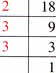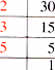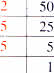Prime factors of  18 = 2×3×3

Prime factors of 30 = 2×3×5

Prime factors of 50 = 2×5×5

Step 2. Rewrite with common factors one below the other.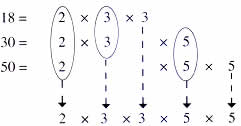Step 3. Multiply: 23×3×5×5 = 450
Thus, LCM of 18, 30 and 50 = 450

### 2. Common Division Method

Find the LCM of 72, 108 and 144 using the common division method.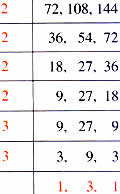LCM = 2×2×2×2×3×3×3
LCM = 432

Step 1. Divide by the smallest prime number that can divide at least one of the numbers. Bring the ones that cannot be divided down as it is.

Step 2. Keep dividing by the Smallest possible prime number till the last row has only prime numbers or numbers that have only 1 has a common factors (co-primes).

Step 3. Multiply all the common factors and all the numbers in the last row to get the LCM.

## चुनौती हिमालय की 5th NCERT CBSE Hindi Rimjhim Ch 18

चुनौती हिमालय की 5th Class NCERT CBSE Hindi Book Rimjhim Chapter 18 प्रश्न: लद्दाख जम्मू-कश्मीर राज्य में …